# Project Repositories

``````(5, 2, 1, 0)
(7, 3, 1, 0)
(11, 4, 1, 0)
(13, 5, 1, 0)
(17, 0, 1, 1)
(19, 1, 1, 1)
(23, 2, 1, 1)
(29, 2, -1, 1)
(31, 1, -1, 1)
(37, 1, 1, 1)
(41, 2, 1, 1)
(43, 3, 1, 1)
(47, 4, 1, 1)
(53, 4, -1, 1)
(59, 4, 1, 1)
(61, 5, 1, 1)
(67, 5, -1, 1)
(71, 4, -1, 1)
(73, 3, -1, 1)
(79, 3, 1, 1)
(83, 4, 1, 1)
(89, 4, -1, 1)
(97, 3, -1, 1)
(101, 2, -1, 1)
(103, 1, -1, 1)
(107, 0, -1, 1)
(109, 5, -1, 0)
(113, 4, -1, 0)
(127, 3, -1, 0)
(131, 2, -1, 0)
(137, 2, 1, 0)
(139, 3, 1, 0)
(149, 4, 1, 0)
(151, 5, 1, 0)
(157, 5, -1, 0)
(163, 5, 1, 0)
(167, 0, 1, 1)
(173, 0, -1, 1)
(179, 0, 1, 1)
(181, 1, 1, 1)
(191, 2, 1, 1)
(193, 3, 1, 1)
(197, 4, 1, 1)
(199, 5, 1, 1)
(211, 5, -1, 1)
(223, 5, 1, 1)
(227, 0, 1, 2)
(229, 1, 1, 2)
(233, 2, 1, 2)
(239, 2, -1, 2)
(241, 1, -1, 2)
(251, 0, -1, 2)
(257, 0, 1, 2)
(263, 0, -1, 2)
(269, 0, 1, 2)
(271, 1, 1, 2)
(277, 1, -1, 2)
(281, 0, -1, 2)
(283, 5, -1, 1)
(293, 4, -1, 1)
(307, 3, -1, 1)
(311, 2, -1, 1)
(313, 1, -1, 1)
(317, 0, -1, 1)
(331, 5, -1, 0)
(337, 5, 1, 0)
(347, 0, 1, 1)
(349, 1, 1, 1)
(353, 2, 1, 1)
(359, 2, -1, 1)
(367, 1, -1, 1)
(373, 1, 1, 1)
(379, 1, -1, 1)
(383, 0, -1, 1)
(389, 0, 1, 1)
(397, 1, 1, 1)
(401, 2, 1, 1)
(409, 3, 1, 1)
(419, 4, 1, 1)
(421, 5, 1, 1)
(431, 0, 1, 2)
(433, 1, 1, 2)
(439, 1, -1, 2)
(443, 0, -1, 2)
(449, 0, 1, 2)
(457, 1, 1, 2)
(461, 2, 1, 2)
(463, 3, 1, 2)
(467, 4, 1, 2)
(479, 4, -1, 2)
(487, 3, -1, 2)
(491, 2, -1, 2)
(499, 1, -1, 2)
(503, 0, -1, 2)
(509, 0, 1, 2)
(521, 0, -1, 2)
(523, 5, -1, 1)
(541, 5, 1, 1)
(547, 5, -1, 1)
(557, 4, -1, 1)
(563, 4, 1, 1)
(569, 4, -1, 1)
(571, 3, -1, 1)
(577, 3, 1, 1)
(587, 4, 1, 1)
(593, 4, -1, 1)
(599, 4, 1, 1)
(601, 5, 1, 1)
(607, 5, -1, 1)
(613, 5, 1, 1)
(617, 0, 1, 2)
(619, 1, 1, 2)
(631, 1, -1, 2)
(641, 0, -1, 2)
(643, 5, -1, 1)
(647, 4, -1, 1)
(653, 4, 1, 1)
(659, 4, -1, 1)
(661, 3, -1, 1)
(673, 3, 1, 1)
(677, 4, 1, 1)
(683, 4, -1, 1)
(691, 3, -1, 1)
(701, 2, -1, 1)
(709, 1, -1, 1)
(719, 0, -1, 1)
(727, 5, -1, 0)
(733, 5, 1, 0)
(739, 5, -1, 0)
(743, 4, -1, 0)
(751, 3, -1, 0)
(757, 3, 1, 0)
(761, 4, 1, 0)
(769, 5, 1, 0)
(773, 0, 1, 1)
(787, 1, 1, 1)
(797, 2, 1, 1)
(809, 2, -1, 1)
(811, 1, -1, 1)
(821, 0, -1, 1)
(823, 5, -1, 0)
(827, 4, -1, 0)
(829, 3, -1, 0)
(839, 2, -1, 0)
(853, 1, -1, 0)
(857, 0, -1, 0)
(859, 5, -1, -1)
(863, 4, -1, -1)
(877, 3, -1, -1)
(881, 2, -1, -1)
(883, 1, -1, -1)
(887, 0, -1, -1)
(907, 5, -1, -2)
(911, 4, -1, -2)
(919, 3, -1, -2)
(929, 2, -1, -2)
(937, 1, -1, -2)
(941, 0, -1, -2)
(947, 0, 1, -2)
(953, 0, -1, -2)
(967, 5, -1, -3)
(971, 4, -1, -3)
(977, 4, 1, -3)
(983, 4, -1, -3)
(991, 3, -1, -3)
(997, 3, 1, -3)
``````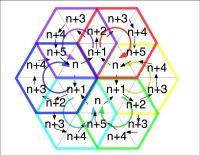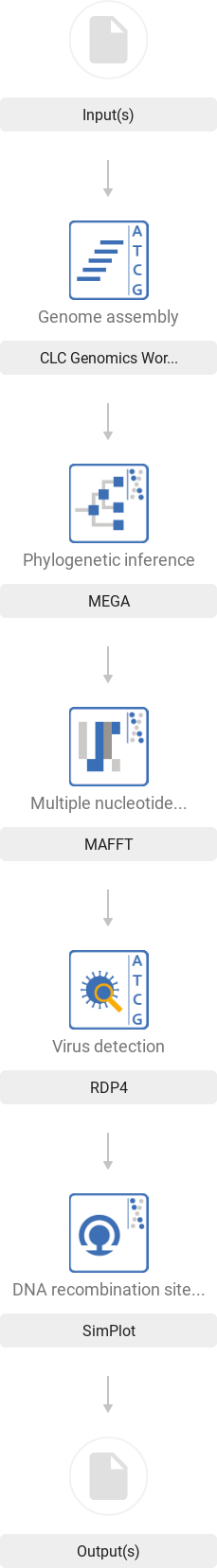139 = 34th prime =(2x17)th prime

``````Scheme 13:9
===========
(1){1}-7:   7'
(1){8}-13:  6‘
(1)14-{19}: 6‘
------------- 6+6 -------
(2)20-24:   5'           |
(2)25-{29}: 5'           |
------------  5+5 -------
(3)30-36:   7:{70,30,10²}|
------------             |
(4)37-48:   12• ---      |
(5)49-59:   11°    |     |
--}30° 30•   |
(6)60-78:   19°    |     |
(7)79-96:   18• ---      |
--------------           |
(8)97-109:  13           |
(9)110-139:{30}=5x6 <--x-- (129/17-139/27)
--
{43}
``````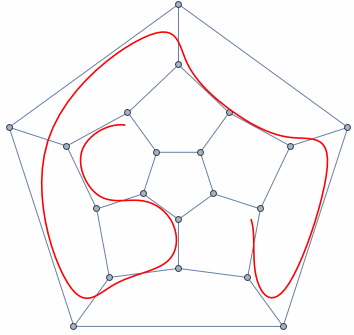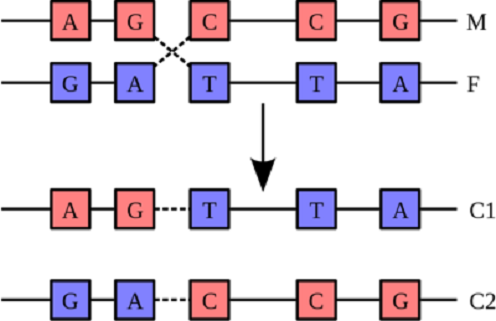``````M: 6® = (2,{3}), ({29,30,31,32}) --> 2,89+29,3 = 289+329 = 618 (main)
F: 6'® = (40,41), (43,44,45,46) --> 30+30+10+10+10+10 = 60+40 (user)
C1: 10°® = 3*®+3*®+4® = (7,13,19),(20,27,36),({38,42,50,68}) --> 200 (main)
C2: 7® = 5®+2® = 1®+4*®+2*® = 1®+6*® = 10,(11,12,14,15,26,28) --> 168 (user)
``````

π (Φ (329 + 289)) = π (Φ x 618) = π (1000) = 168

``````1st layer:
It has a total of 1000 numbers
Total primes = π(1000) = 168 primes

2nd layer:
It will start by π(168)+1 as the 40th prime
It has 100x100 numbers or π(π(10000)) = 201 primes
Total cum primes = 168 + (201-40) = 168+161 = 329 primes

3rd layer:
Behave the same as 2nd layer which has a total of 329 primes
The primes will start by π(π(π(1000th prime)))+1 as the 40th prime
This 1000 primes will become 1000 numbers by 1st layer of the next level
Total of all primes = 329 + (329-40) = 329+289 = 618 = 619-1 = 619 primes - Δ1
``````

## Pinned Repo

### User repos

``````# pinned repos
# https://dev.to/thomasaudo/get-started-with-github-grapql-api--1g8b
echo -e "\n\$hr\nPINNED  REPOSITORIES\n\$hr"
AUTH="Authorization: bearer \$JEKYLL_GITHUB_TOKEN"
curl -L -X POST "\${GITHUB_GRAPHQL_URL}" -H "\$AUTH" \
{\n pinnedItems(first: 6, types: REPOSITORY) {\n nodes {\n ... on Repository \
{\n name\n }\n }\n }\n }\n}"'

================================================================================
PINNED  REPOSITORIES
================================================================================
% Total    % Received % Xferd  Average Speed   Time    Time     Time  Current

0     0    0     0    0     0      0      0 --:--:-- --:--:-- --:--:--     0
100   292  100   150  100   142   1171   1109 --:--:-- --:--:-- --:--:--  2281

{"data":{"user":{"pinnedItems":{"nodes":[{"name":"maps"},{"name":"feed"},
{"name":"lexer"},{"name":"parser"},{"name":"syntax"},{"name":"grammar"}]}}}}
``````

### Organization (View as: Public

GitHub graphQL API multiple queries on organizations &

``````curl -L -X POST "\${GITHUB_GRAPHQL_URL}" -H "\$AUTH" \
{\n pinnedItems(first: 6, types: REPOSITORY) {\n nodes {\n ... on Repository \
{\n name\n }\n }\n }\n }\n}"'

================================================================================
PINNED  REPOSITORIES
================================================================================
% Total    % Received % Xferd  Average Speed   Time    Time     Time  Current

0     0    0     0    0     0      0      0 --:--:-- --:--:-- --:--:--     0
100   292  100   150  100   142   1171   1109 --:--:-- --:--:-- --:--:--  2281

{"data":{"organization":{"pinnedItems":{"nodes":[{"name":"classifier"},
{"name":"domJSON"},{"name":"openoffice"},{"name":"landing-page-theme"},
{"name":"asciidoc"},{"name":"recommendations-ai"}]}}}}
``````

1 instance + 7 blocks + 29 flats + 77 rooms = 114 objects

``````True Prime Pairs:
(5,7), (11,13), (17,19)

-----+-----+-----+-----+-----+     -----------------------------------------------
{786}| 1,2 |  2  | 2,3 | 3,4 | {19}                                          |
-----+-----+-----+-----+-----+                                               |
{86}|  4  | 4,5 | 5,6 |{6,7}| 17                                        Base Zone
+-----+-----+-----+-----+                                               |
{78}|{7,8}| 8,9 | 12 (M dan F) ----> Δ                                      |
+-----+-----+-----+                                               -----------
{67}| 9,11|11,12|12,14| 11 <----------- Mid Zone                            |
----+-----+-----+-----+-----+                                               |
{6}|15,16|17,18|18,20|21,22| 19                                      Mirror Zone
+-----+-----+-----+-----+                                               |
{8}|23,25|25,27|27,29| 18                                                  |
+-----+-----+-----+-----+-----+-----+-----+-----+-------+         -----------
{7}|29,33|33,36|36,39|39,41|41,45|46,51|51,57|58,66|{67,77}| 43 (C1 dan C2)<---Δ
-----+-----+-----+-----+-----+-----+-----+-----+-----+-------+         -----------
|  1     2     3  |   4     5     6 |   7     8      9  |
|------ 29' ------|--------------- 139' ----------------|
|------ 102¨ -----|---------------  66¨ ----------------|
``````

114 = 102 + 66 - 29 - 25 = 6 + (6x6) + 6 x (6+6) = 6 x (6+6) + 6 + (6x6) = 25 + 89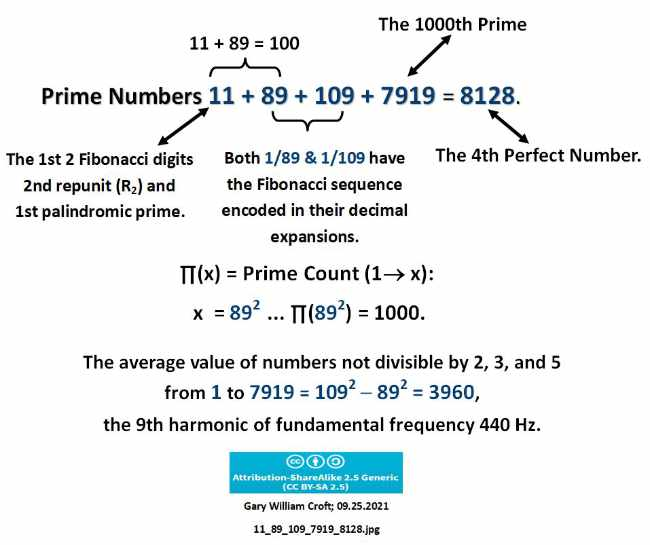6 x 114 - 30 - 30 - 5 = 619 = 6 x 19 = 114th prime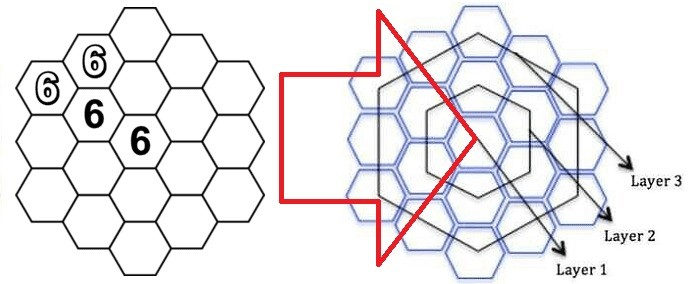π(1000) + 1000/Φ = 168 + 618 = (7x71) + (17x17) = 786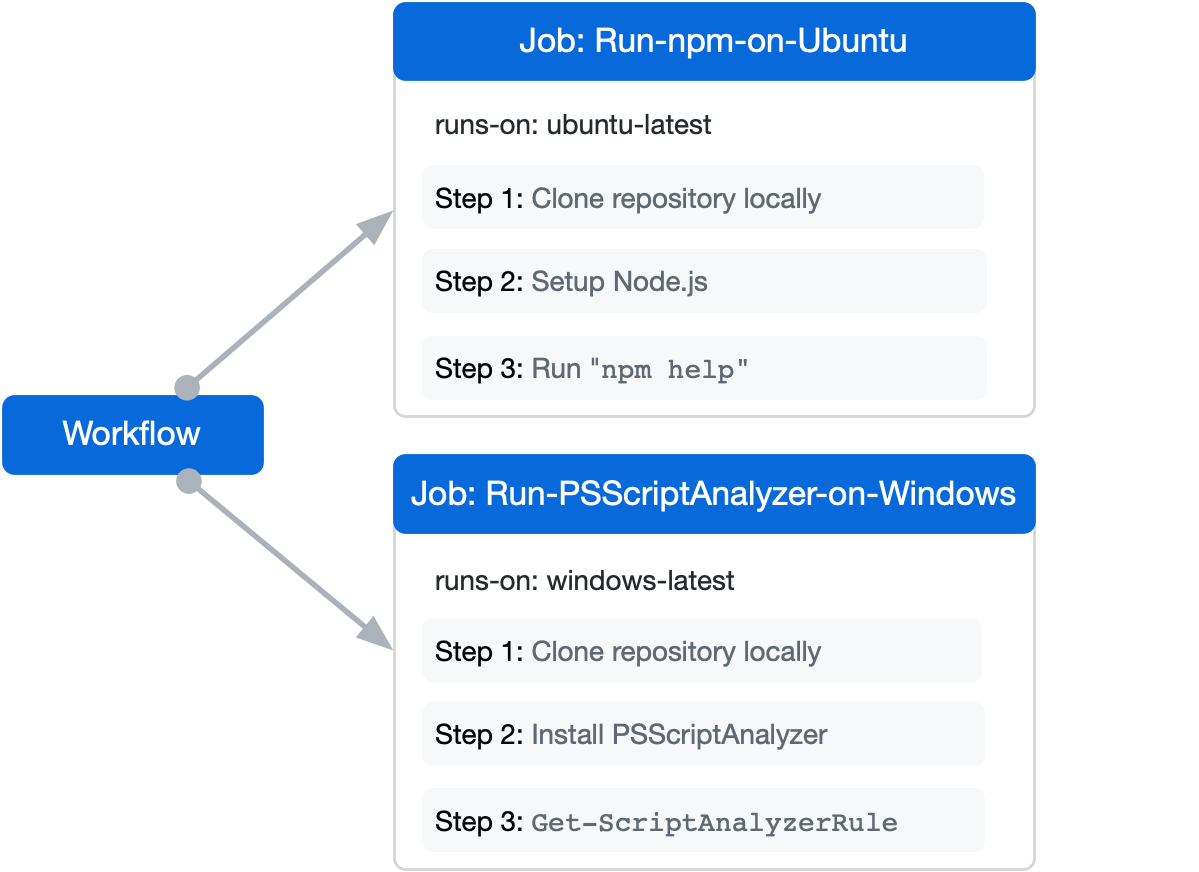To make it easier to develop a program following a model, we divide the object by placing it into a smaller objects. We do this division by adopting the OOP (Object Oriented Programming) which is an object-oriented programming method.

139 + 286 + 114 + 247 + 157 + 786 = 786 + 157 + 786 = 1729 = 7 x 13 x 19168 + 329 + 289 = 168 + 618 = 786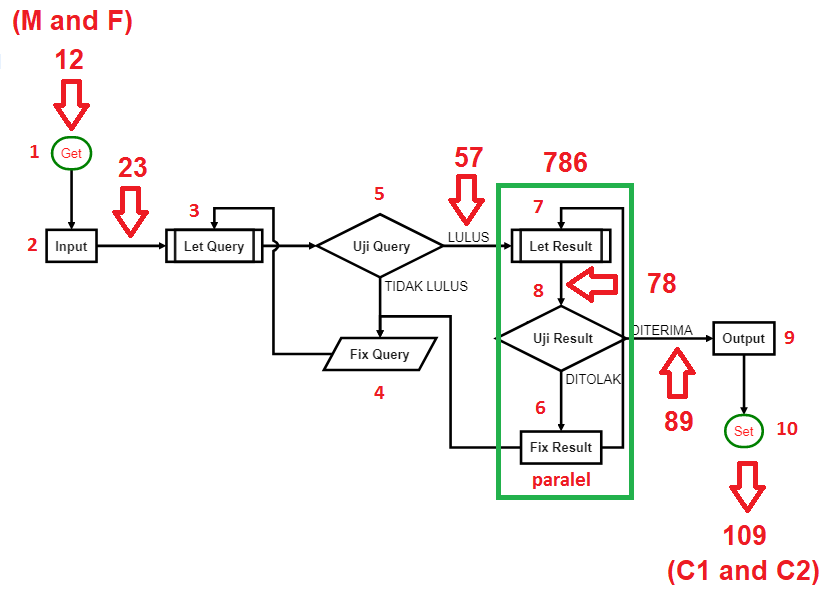Using this method then out of bilateray way of 19 vs 18 we could get in to Scheme-33. By a decomposition the subject is divided into six (6) parts, each part is further regrouped in to 19 sub-parts so finaly we got π(1000/Φ)=π(618)=114 objects.

d(43,71,114) = d(7,8,6) » 786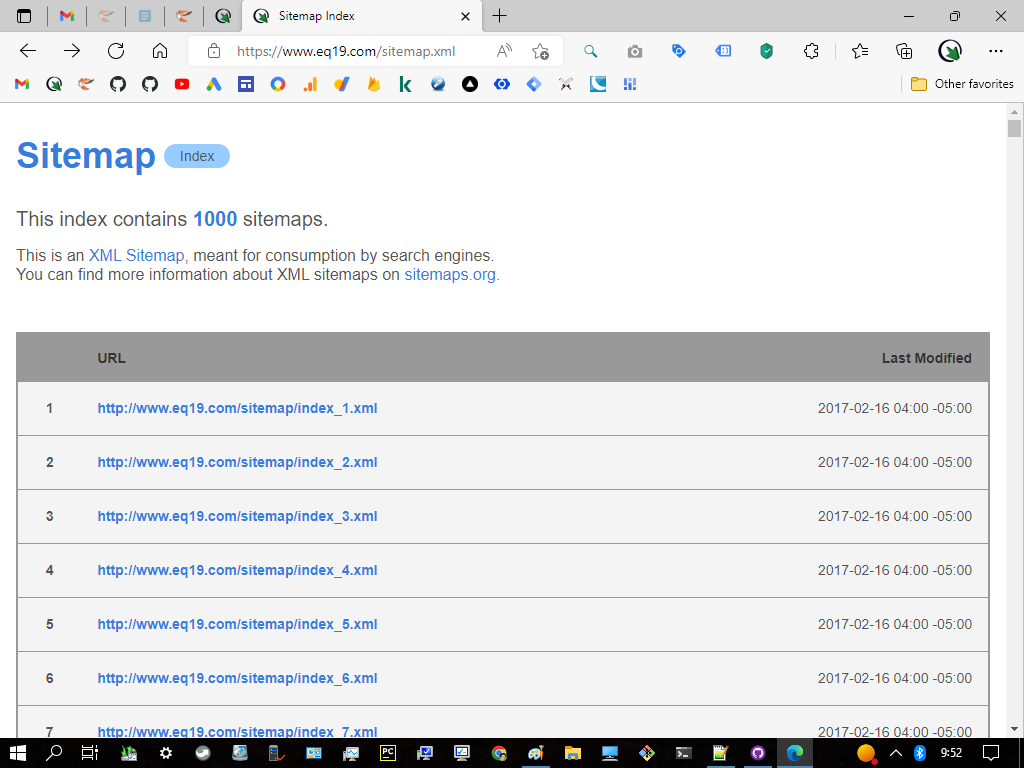By defining the pattern on each individual numbers against homogeneous sorting out of these 114 objects then we finally come to a scale called MEC30 which is based on the beauty of Euler's identity.

Mathematics writer Constance Reid has opined that Euler's identity is the most famous formula in all mathematics. And Benjamin Peirce, a 19th-century American philosopher, mathematician, and professor at Harvard University, after proving Euler's identity during a lecture, stated that the identity "is absolutely paradoxical; we cannot understand it, and we don't know what it means, but we have proved it, and therefore we know it must be the truth" (Wikipedia)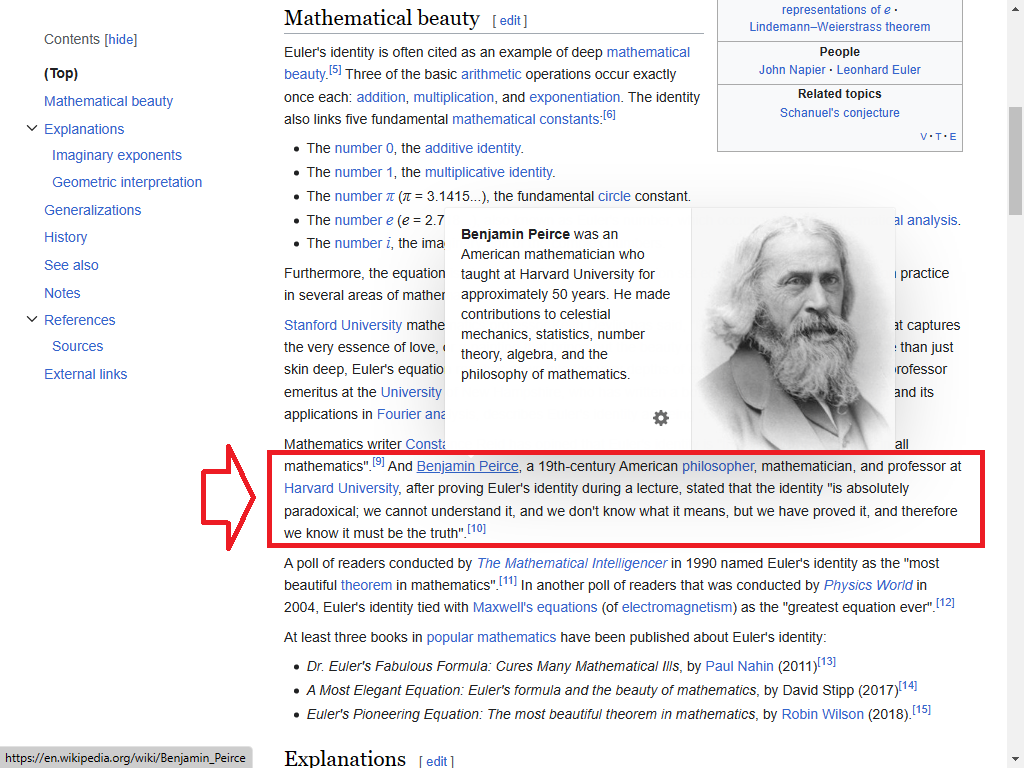Although this may seem very fast, a much more sophisticated method, combined with a very powerful computer, is necessary to find very large primes. Despite there are many studies and papers it is still an important open problem today.

The Millennium Prize Problems are seven problems in mathematics that were stated by the Clay Mathematics Institute in 2000. Currently, six of the problems remain unsolved (Wikipedia).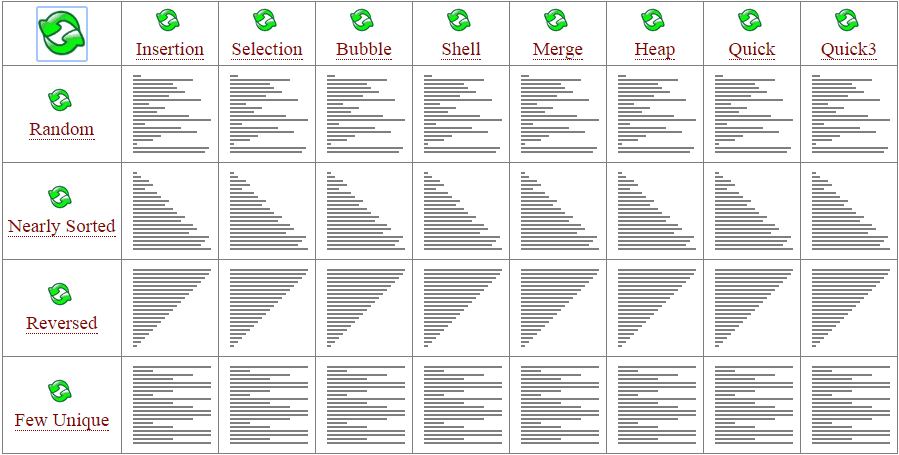In the next section we will discuss about algorithms in applications and their relation to the distribution of prime numbers.